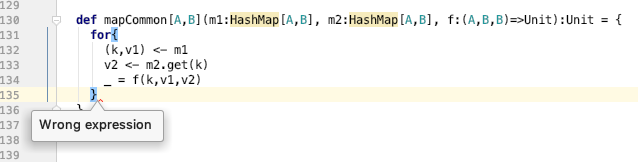# Map over common keys

I’m looking an efficient way to identify common keys of two `HashMap` objects. One way would be to call `hashKeys` on both and calculate the intersection. However this has n^2 complexity. The other way it to iterate through one, and for each key use `get` to determine whether the key is in the second hash.

Here is the best way I can find to do this. It’s ugly because I’m simply returning `None` from the flatMap to avoid accumulating a sequence.

``````  def mapCommon[A,B](m1:HashMap[A,B], m2:HashMap[A,B], f:(A,B,B)=>Unit):Unit = {
m1.flatMap { case (k, v1) =>
m2.get(k).flatMap { v2 =>
f(k, v1, v2)
None
}
}
}
``````

An alternate implementation which unfortunately accumulates a sequence just to throw it away is the following. It is more readable, in my opinion, but inefficient.

``````  def mapCommon_alternate[A,B](m1:HashMap[A,B], m2:HashMap[A,B], f:(A,B,B)=>Unit):Unit = {
for{
(k,v1) <- m1
v2 <- m2.get(k)
} yield f(k,v1,v2)
}
``````

it would be nice If I could ask the for expander to expand the inner most loop into a `flatMap` rather than a `map`, then I could do the following.

``````  def mapCommon_alternate[A,B](m1:HashMap[A,B], m2:HashMap[A,B], f:(A,B,B)=>Unit):Unit = {
for{
(k,v1) <- m1
v2 <- m2.get(k)
_ = f(k,v1,v2)
} yield None // flatMap me rather than map
}
``````

I think I’m missing something – what’s the benefit of the inner `flatMap`? If you just want to return `Unit` anyway, why not just leave off the `yield`, and have the whole thing `foreach` instead? I assume that `f()` is some sort of side-effect that does what you’re actually looking for, so your second formulation without the `yield` looks both clearest and most efficient…

Here’s what I get if I leave off the yieldHowever, the following does seem to work, and passes my test cases.

``````  def mapCommon[A,B](m1:HashMap[A,B], m2:HashMap[A,B], f:(A,B,B)=>Unit):Unit = {
for{
(k,v1) <- m1
v2 <- m2.get(k)
} f(k,v1,v2)
}
``````

Correct – the latter is what I meant: literally just drop the `yield` keyword.

`yield` is one of those crucial subtleties: it’s optional, but totally changes the semantics of a `for` comprehension. With `yield`, everything translates to map/flatMap, and it’s a central part of functional programming. Without `yield`, everything translates to `foreach`, and you have a side-effecting imperative program instead.

Idiomatic Scala code tends to lean functional, so you’ll usually see the `yield` there. But when you want to do side-effects instead, it’s usually more efficient to omit it, and everything will return `Unit` all the way down…

1 Like

That’s really nice syntactically. here is what the code reduces to using the for comprehension. In my opinion, it is very subtle the interaction between hash.get which returns an `Option` and `flatMap` on an option. The new version risks being too terse. It’s not clear anymore that we’re iterating over the common keys of two hash maps. I’m tempted to put back in some type annotations, just to make what’s happening clearer to the human.

``````  def reduceOne(posCount: Int, addFunction: HashUpdateFunction, removeFunction: HashUpdateFunction): Unit = {
val posCountDec = posCount - 1
(hash.get(posCount), hash.get(posCountDec)) match {
case (None, _) => Unit
case (_, None) => Unit
case (Some(lengthHashA), Some(lengthHashB)) =>
for{
(length,rectHashA) <- lengthHashA
rectHashB <- lengthHashB.get(length)
(rectified,cla) <- rectHashA
clb <- rectHashB.get(rectified)
} crossCompatiblize(cla, clb, posCount, length, rectified, removeFunction, addFunction)
}
}
``````

The original is here. Worked, but was really hard to figure out what it does.

``````  def reduceOne(posCount: Int, addFunction: HashUpdateFunction, removeFunction: HashUpdateFunction): Unit = {
val posCountDec = posCount - 1
(hash.get(posCount), hash.get(posCountDec)) match {
case (None, _) => Unit
case (_, None) => Unit
case (Some(lengthHashA), Some(lengthHashB)) =>
for {(length, rectHashA) <- lengthHashA} {
lengthHashB.get(length) match {
case None => Unit
case Some(rectHashB) =>
rectHashA.keySet.intersect(rectHashB.keySet).foreach { rectified => {
val cla = rectHashA(rectified)
val clb = rectHashB(rectified)
crossCompatiblize(cla, clb, posCount, length, rectified, removeFunction, addFunction)
}
}
}
}
}
}
``````

What makes you think that the default implementation is O(n^2)?

The other way it to iterate through one, and for each key use `get` to determine whether the key is in the second hash.

That’s exactly what the default implementation does:

``````def intersect(that: Set[A]): C = this.filter(that)
``````

Yes, I guess you’re right indeed. my original code

``````rectHashA.keySet.intersect(rectHashB.keySet)
``````

was probably not as inefficient as I first thought. Thanks for pointing that out.